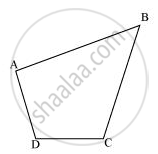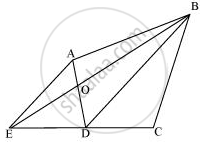Share

# A villager Itwaari has a plot of land of the shape of a quadrilateral. The Gram Panchayat of the village decided to take over some portion of his plot from one of the corners to construct a Health Centre. - CBSE Class 9 - Mathematics

ConceptTriangles on the Same Base and Between the Same Parallels

#### Question

A villager Itwaari has a plot of land of the shape of a quadrilateral. The Gram Panchayat of the village decided to take over some portion of his plot from one of the corners to construct a Health Centre. Itwaari agrees to the above proposal with the condition that he should be given equal amount of land in lieu of his land adjoining his plot so as to form a triangular plot. Explain how this proposal will be implemented.

#### SolutionLet quadrilateral ABCD be the original shape of the field.

The proposal may be implemented as follows.

Join diagonal BD and draw a line parallel to BD through point A. Let it meet

the extended side CD of ABCD at point E. Join BE and AD. Let them intersect each other at O. Then, portion ΔAOB can be cut from the original field so that the new shape of the field will be ΔBCE. (See figure)

We have to prove that the area of ΔAOB (portion that was cut so as to construct Health Centre) is equal to the area of ΔDEO (portion added to the field so as to make the area of the new field so formed equal to the area of the original field)It can be observed that ΔDEB and ΔDAB lie on the same base BD and are between the same parallels BD and AE.

∴ Area (ΔDEB) = Area (ΔDAB)

⇒ Area (ΔDEB) − Area (ΔDOB) = Area (ΔDAB) − Area (ΔDOB)

⇒ Area (ΔDEO) = Area (ΔAOB)

Is there an error in this question or solution?

#### APPEARS IN

NCERT Solution for Mathematics Class 9 (2018 to Current)
Chapter 9: Areas of Parallelograms and Triangles
Ex. 9.30 | Q: 12 | Page no. 164

#### Video TutorialsVIEW ALL 

Solution A villager Itwaari has a plot of land of the shape of a quadrilateral. The Gram Panchayat of the village decided to take over some portion of his plot from one of the corners to construct a Health Centre. Concept: Triangles on the Same Base and Between the Same Parallels.
S